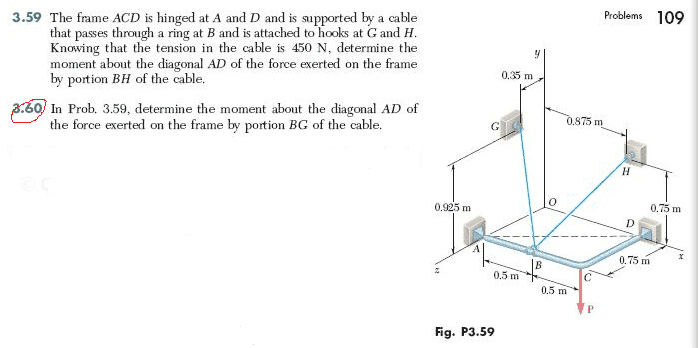# Statics Problem, Moment About an Axis

## Homework Statement

Problem 3.60:## Homework Equations

$M_{AD}= \begin{vmatrix} \lambda_x & \lambda_y & \lambda_z \\ x_{B/A} & y_{B/A} & z_{B/A} \\ F_x & F_y & F_z & \end{vmatrix}$

## The Attempt at a Solution

Looking at the figure, these are the unit vector components for line AD:

$\lambda_x=1 \ m \\ \lambda_y= 0 \ m \\ \lambda_z = -0.75 \ m$

And these are the coordinates for point B where the force is applied:

$x_{B/A}=0.5 \ m \\ y_{B/A}= 0 \ m \\ z_{B/A} = 0 \ m$

The force vector for the tension in BG:
$\mathbf{T_{BG}}= 450*(\frac{-0.5\hat{i}+0.925\hat{j}-0.4\hat{k}}{1.125})= -200\hat{i}+370\hat{j}-160\hat{k} \ N$

Plugging these values into the matrix above, I get -138.75 N*m, but the answer is supposed to be -111.0 N*m

Edit: Changed the mistake I made in the second row. Still getting the wrong answer.

Last edited:

## Answers and Replies

SteamKing
Staff Emeritus
Science Advisor
Homework Helper
Shouldn't λx^2+λy^2+λz^2 = 1 if these are the components of a unit vector?

Shouldn't λx^2+λy^2+λz^2 = 1 if these are the components of a unit vector?

Yes, I actually just figured it out. I had messed up when I took the unit vector components and didn't divide by the magnitude.
Is there a way I can close this thread?

berkeman
Mentor
We don't delete threads that have responses. Glad that you figured it out.